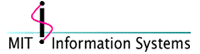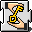# Macintosh DevelopmentDES Library API Functions
 `int` `des_cbc_encrypt (` ```des_cblock *in, des_cblock *out, long length, des_key_schedule schedule, des_cblock ivec, int encrypt );``` `void` `des_3cbc_encrypt (` ```des_cblock *in, des_cblock *out, long length, des_key_schedule ks1, des_key_schedule ks2, des_key_schedule ks3, des_cblock ivec, int encrypt );```

`des_cbc_encrypt()` encrypts/decrypts using the cipher-blockchaining mode of DES.

If the `encrypt` argument is nonzero, the routine cipher-block-chain encrypts the cleartext data pointed to by the `in` argument into the ciphertext pointed to by the `out` argument, using the key schedule provided by the `schedule` argument, and initialization vector provided by the `ivec` argument. If the `length` argument is not an integral multiple of eight bytes, the last block is copied to a temp and zero filled (highest addresses). `out` is ALWAYS an integral multiple of eight bytes.

If `encrypt` is zero, the routine cipher-block chain decrypts the (now) ciphertext data pointed to by the `in` argument into (now) cleartext pointed to by the `out` argument using the key schedule provided by the `schedule` argument, and initialization vector provided by the `ivec` argument. Decryption ALWAYS operates on integral multiples of 8 bytes, so it will round the length provided up to the appropriate multiple. Consequently, it will always produce the rounded-up number of bytes of output cleartext. The application must determine if the output cleartext was zero-padded due to original cleartext lengths that were not integral multiples of 8.

`des_3cbc_encrypt()` does the same thing as `des_cbc_encrypt()`, except using Kerberos 5 triple DES. In order to have enough bits of key, `des_3cbc_encrypt()` requires 3 key schedules.

No errors or meaningful values are returned by either function.

 `unsigned long` `des_cbc_cksum (` ```des_cblock *in, des_cblock *out, long length, des_key_schedule schedule, des_cblock *ivec );```

`des_cbc_cksum()` produces an 8 byte cryptographic checksum by cipher-block-chain encrypting the cleartext data pointed to by the `in` argument. All of the ciphertext output is discarded, except the last 8-byte ciphertext block, which is written into the area pointed to by the `out` argument. It uses the key schedule, provided by the `schedule` argument and initialization vector provided by the `ivec` argument. If the length argument is not an integral multiple of eight bytes, the last cleartext block is copied to a temp and zero filled (highest addresses). The output is ALWAYS eight bytes.

The routine also returns an unsigned long, which is the last (highest address) half of the 8 byte checksum computed.

 `int` `des_ecb_encrypt (` ```des_cblock *in, des_cblock *out, des_key_schedule schedule, int encrypt );``` `void` `des_3ebc_encrypt (` ```des_cblock *in, des_cblock *out, des_key_schedule ks1, des_key_schedule ks2, des_key_schedule ks3, int encrypt );```

`des_ecb_encrypt()` is the basic DES encryption routine that encrypts or decrypts a single 8-byte block in electronic code book mode. It always transforms the input data, pointed to by `in`, into the output data, pointed to by the `out` argument.

If the `encrypt` argument is non-zero, the `in` (cleartext) is encrypted into the `out` (ciphertext) using the key schedule specified by the `schedule` argument, previously set via `des_set_key()`.

If `encrypt` is zero, the `in` (now ciphertext) is decrypted into the `out` (now cleartext).

Input and output may overlap. No errors or meaningful values are returned by either function.

 `int` `des_pcbc_encrypt (` ```des_cblock *in, des_cblock *out, long length, des_key_schedule schedule, des_cblock *ivec, int encrypt );```

`des_pcbc_encrypt()` encrypts/decrypts using a modified block chaining mode. Its calling sequence is identical to `des_cbc_encrypt()`. It differs in its error propagation characteristics.

`des_pcbc_encrypt()` is recommended for most encryption purposes, in that modification of a single bit of the ciphertext will affect ALL the subsequent (decrypted) cleartext. Similarly, modifying a single bit of the cleartext will affect ALL the subsequent (encrypted) ciphertext. "PCBC" mode, on encryption, "xors" both the cleartext of block N and the ciphertext resulting from block N with the cleartext for block N+1 prior to encrypting block N+1.

No errors or meaningful values are returned by either function.

 `unsigned long` `des_quad_cksum (` ```unsigned char *in, unsigned long *out, long length, int out_count, des_cblock *c_seed );```

`des_quad_cksum()` calculates a manipulation detection code for a message. It is a much faster alternative to the DES-checksum method. No guarantees are offered for its security. Refer to "Message Authentication" R.R. Jueneman, S. M. Matyas, C.H. Meyer IEEE Communications Magazine, Sept 1985 Vol 23 No 9 p 29-40 for more information.

 `int` `des_random_key (` `des_cblock *key );`

`des_random_key()` generates a random DES encryption key (eight bytes), set to odd parity per FIPS specifications. This routine uses the current time, and a counter as a seed for the random number generator. The caller must supply space for the output key, pointed to by argument `key`, then after calling `des_random_key()` should call the `des_set_key()` routine when needed.

No meaningful value is returned. Void is not used for compatibility with other compilers.

 `void` `des_init_random_number_generator (` `des_cblock key );` `void` `des_set_random_generator_seed (` `des_cblock key );` `int` `des_new_random_key (` `des_cblock key );` `void` `des_set_sequence_number (` `des_cblock new_sequence_number );` `void` `des_generate_random_block (` `des_cblock block );`

These functions provide a better interface for creating random keys. By allowing the programmer to specify seeds, and the sequence number, the programmer can control randomness better than with des_random_key().

`des_init_random_number_generator()` takes a secret key possibly shared by a number of servers and uses it to generate a random number stream that is not shared by any of the other servers. It does this by calling `des_set_random_generator_seed()` with the current time to the nearest 1/60th of a second. The resulting stream seed is not useful information for cracking the secret key. Moreover, this routine keeps no copy of the secret key.

`des_new_random_key()` sets `key` to a new random key. The returned key has correct parity and is guaranteed not to be a weak DES key. `des_generate_random_block()` is used to provide the random bits. `des_set_random_generator_seed()` must be at called least once before this routine is called (calling `des_init_random_number_generator()` is sufficient).

`des_set_random_generator_seed()` is used to select a random number stream. The stream that results is totally determined by the passed in key. (I.e., calling this routine again with the same key allows repeating a sequence of random numbers). The key must be a valid DES key: it must have correct parity and not be a weak DES key. Use this function if you do not want the key to be seeded by the current time.

`des_set_sequence_number()` is used to set the sequence number of the current random number stream. This routine may be used to "seek" within the current random number stream. Note that `des_set_random_generator_seed()` resets the sequence number to 0.

`des_generate_random_block()` returns the next random number from the current random number stream. The returned number is 64 bits long. `des_set_random_generator_seed()` must have been called at least once before this routine is called.

 `int` `des_check_key_parity (` `register des_cblock key );` `void` `des_fixup_key_parity (` `register des_cblock key );`

`des_check_key_parity()` returns true if key has the correct DES parity, false if not. Correct parity is defined as odd parity per byte; parity is bits 8,16,...64 in DES order, implies 0, 8, 16, ... in VAX byte order.

`des_fixup_key_parity()` modifies a key so that it has the correct parity for DES.

 `void` `des_is_weak_key (` `des_cblock key );`

`des_is_weak_key()` returns true if key is a [semi-]weak DES key, false if the key is strong. You should not use weak keys.

 `int` `des_string_to_key (` ```char *str, des_cblock key );``` `int` `afs_des_string_to_key (` ```char *str, char *cell, des_cblock key );```

`des_string_to_key()` and `afs_des_string_to_key()` convert an arbitrary length null-terminated string to an 8 byte DES key, with odd byte parity, per FIPS specification. A one-way function is used to convert the string to a key, making it very difficult to reconstruct the string from the key. The `str` argument is a pointer to the string, and `key` should point to a des_cblock supplied by the caller to receive the generated key. `afs_des_string_to_key()` also requires `cell`: the name of the AFS cell to authenticate to.

Note: `des_string_to_key()` is the MIT string to key function and `afs_des_string_to_key()` is the AFS string to key function. If you need to use a different string to key than either the MIT string to key or the AFS string to key, you will have to implement it yourself..

No meaningful value is returned. Void is not used for compatibility with other compilers.

 `int` `des_set_key (` ```des_cblock *key, des_key_schedule schedule );```

`des_set_key()` calculates a key schedule from all eight bytes of the input key, pointed to by the `key` argument, and outputs the schedule into the `des_key_schedule` indicated by the `schedule` argument. Make sure to pass a valid eight byte key; no padding is done. The key schedule may then be used in subsequent encryption/decryption/checksum operations. Many key schedules may be cached for later use. The user is responsible to clear keys and schedules as soon as no longer needed, to prevent their disclosure.

The routine also checks the key parity, and returns a zero if the key parity is correct (odd), a -1 indicating a key parity error, or a -2 indicating use of an illegal weak key. If an error is returned, the key schedule was not created.

 `int` `make_key_sched (` ```des_cblock *key, des_key_schedule schedule );``` `int` `des_key_sched (` ```des_cblock k, des_key_schedule schedule );```

`make_key_sched()` computes the DES key schedule given a key

`des_key_sched()` does the same thing as `make_key_sched()` except it also checks to make sure the key is not weak and has the correct parity. It returns a zero if successful, a -1 indicating a key parity error, or a -2 indicating use of a weak key.

 `char *` `des_crypt (` ```const char *buf, const char *salt );``` `char *` `des_fcrypt (` ```const char *buf, const char *salt, char *ret );```

`des_crypt()` is effectively the UNIX crypt function. `buf` is the NULL-terminated C string to encrypt and `salt` is the two character salt for the crypt cipher. `des_crypt()` returns the encrypted string. You should copy this string before calling `des_crypt()` again.

`des_fcrypt()` is the same as `des_crypt()`, except that the encrypted string is placed in `ret`. You must allocate enough space for the encrypted string in `ret` (14 bytes will be sufficient).

 `int` `des_read_password (` ```des_cblock *k, char *prompt, int verify );``` `int` `des_read_pw_string (` ```char *s, int max, char *prompt, int verify );```

`des_read_password()` writes the string specified by `prompt` to the standard output, turns off echo (if possible) and reads an input string from standard input until terminated with a newline. If `verify` is non-zero, it prompts and reads input again, for use in applications such as changing a password; both versions are compared, and the input is requested repeatedly until they match. Then `des_read_password()` converts the input string into a valid DES key, internally using `des_string_to_key()`. The newly created key is copied to the area pointed to by the `key` argument. `des_read_password()` returns a zero if no errors occurred, or a -1 indicating that an error occurred trying to manipulate the terminal echo.

`des_read_pw_string()` reads in a password without calling `des_string_to_key()` (`des_read_password()` calls `des_read_pw_string()` internally).

NOTE! `des_read_password()` and `des_read_pw_string()` are only for use with the Metrowerks SIOUX command line interfaces in Kerberos Support Library. If you are not using SIOUX, read in the password yourself and call `des_string_to_key()` manually. Normally you will only use these functions for testing.

 `void` `des_cblock_print_file (` ```des_cblock *x, FILE *fp );```

`des_cblock_print_file()` is a debugging function used to print out a key to a given FILE descriptor. `des_cblock_print_file()` is only for use with the Metrowerks SIOUX command line interfaces in MIT Support Library.

Questions or comments? Send mail to macdev@mit.edu
Last updated on \$Date: 2003/11/19 20:42:25 \$
Last modified by \$Author: smcguire \$